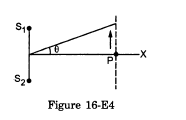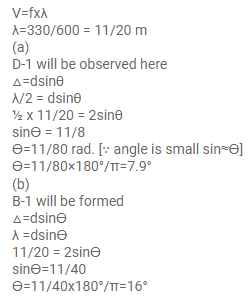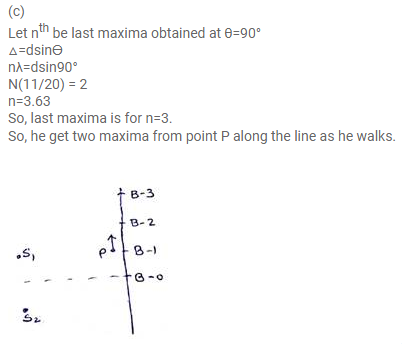# Two speakers S1 and S2, driven by the same amplifier,

Question:

Two speakers $S_{1}$ and $S_{2}$, driven by the same amplifier, are placed at $y=1.0 \mathrm{~m}$ and $y=-1.0 \mathrm{~m}$. The speakers vibrate in phase at $600 \mathrm{~Hz}$. A man stands at a point on the $X$-axis at a very large distance from the origin and starts moving parallel to the $Y$ axis. The speed of sound in air is $330 \mathrm{~m} \mathrm{~s}^{-1}$. (a) At what angle $\theta$ will the intensity of sound drop to a minimum for the first time? (b) At what angle will he hear a maximum of sound intensity for the first time? (c) If he continues to walk along the line, how many more can he hear?Solution: